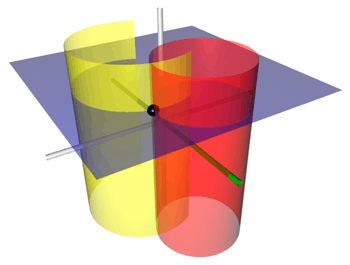# Bipolar cylindrical coordinatesCoordinate surfaces of the bipolar cylindrical coordinates. The yellow crescent corresponds to σ, whereas the red tube corresponds to τ and the blue plane corresponds to z=1. The three surfaces intersect at the point P (shown as a black sphere).

Bipolar cylindrical coordinates are a three-dimensional orthogonal coordinate system that results from projecting the two-dimensional bipolar coordinate system in the perpendicular-direction. The two lines of fociandof the projected Apollonian circles are generally taken to be defined byand, respectively, (and by) in the Cartesian coordinate system.

The term "bipolar" is often used to describe other curves having two singular points (foci), such as ellipses, hyperbolas, and Cassini ovals. However, the term bipolar coordinates is never used to describe coordinates associated with those curves, e.g., elliptic coordinates.

## Basic definition

The most common definition of bipolar cylindrical coordinatesiswhere thecoordinate of a pointequals the angleand thecoordinate equals the natural logarithm of the ratio of the distancesandto the focal lines(Recall that the focal linesandare located atand, respectively.)

Surfaces of constantcorrespond to cylinders of different radiithat all pass through the focal lines and are not concentric. The surfaces of constantare non-intersecting cylinders of different radiithat surround the focal lines but again are not concentric. The focal lines and all these cylinders are parallel to the-axis (the direction of projection). In theplane, the centers of the constant-and constant-cylinders lie on theandaxes, respectively.

## Scale factors

The scale factors for the bipolar coordinatesandare equalwhereas the remaining scale factor. Thus, the infinitesimal volume element equalsand the Laplacian is given byOther differential operators such asandcan be expressed in the coordinatesby substituting the scale factors into the general formulae found in orthogonal coordinates.

## Applications

The classic applications of bipolar coordinates are in solving partial differential equations, e.g., Laplace's equation or the Helmholtz equation, for which bipolar coordinates allow a separation of variables (in 2D). A typical example would be the electric field surrounding two parallel cylindrical conductors.

## Bibliography

• Margenau H, Murphy GM (1956). The Mathematics of Physics and Chemistry. New York: D. van Nostrand. pp. 187190. LCCN 55010911.
• Korn GA, Korn TM (1961). Mathematical Handbook for Scientists and Engineers. New York: McGraw-Hill. p. 182. LCCN 59014456. ASIN B0000CKZX7.
• Moon P, Spencer DE (1988). "Conical Coordinates (r, θ, λ)". Field Theory Handbook, Including Coordinate Systems, Differential Equations, and Their Solutions (corrected 2nd ed., 3rd print ed.). New York: Springer-Verlag. unknown. ISBN 978-0-387-18430-2.